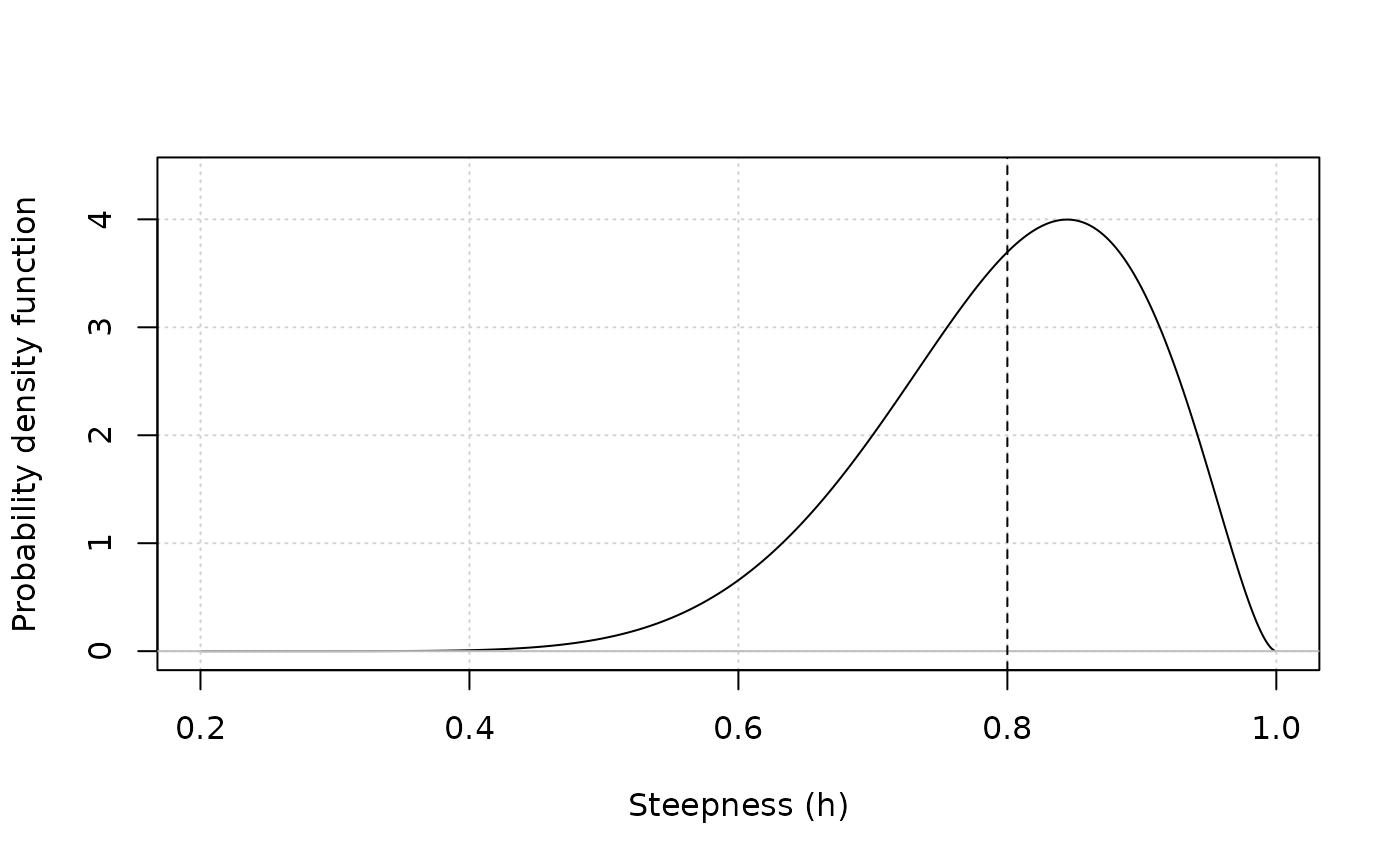Plots the probability distribution function of steepness from the mean and standard deviation.

plot_steepness(
m,
sd,
is_transform = FALSE,
SR = c("BH", "Ricker"),
color = "black"
)

## Arguments

m

The mean of the distribution (vectorized).

sd

The standard deviation of the distribution (vectorized).

is_transform

Logical, whether the mean and standard deviation are in normal space (FALSE) or transformed space.

SR

The stock recruitment relationship (determines the range and, if relevant, transformation of steepness).

color

A vector of colors.

## Value

A plot of the probability distribution function. Vertical dotted line indicates mean of distribution.

## Note

The function samples from a beta distribution with parameters alpha and beta that are converted from the mean and standard deviation. Then, the distribution is transformed from 0 - 1 to 0.2 - 1.

plot_lognormalvar plot_betavar

Q. Huynh

## Examples

mu <- 0.8
stddev <- 0.1
plot_steepness(mu, stddev)